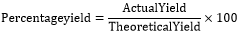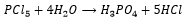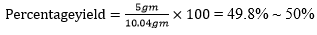# Theoretical Yield Formula

## Theoretical Yield Formula - Solved Examples & Practice Questions

In theory, we can always predict the amount of desired product that will be formed at the end of a chemical reaction. Assuming that the reaction will go to completion we can predict this amount of product from the stoichiometric coefficients of the balanced chemical equation. But in general, due to several factors ranging from the purity of the chemicals used to maintaining the perfect reaction condition, the yield of the product obtained is always less than the desired value.

So after performing an experiment, we follow a protocol to report the yield of product in percentage and compare it with theoretical yield (desired yield) to approve the usage and efficiency of a reaction.
In order to calculate the percentage yield of the product we use the following formula:Let us understand the application of this formula in the following example.

Solved example: (i) For the balanced equation shown below, if 93.8 grams of PCl5 were reacted with 20.3 grams of H2O, how many grams of H3PO4 would be produced?

(ii) If the amount of H3PO4 was found to be 20 gm instead of the expected value, what will be the percentage yield?Solution:
(i) P = 31; Cl = 35.5; H = 1; O = 16;
molar mass of PCl5 = 31* 1 + 35.5 * 5 = 208.5 grams/mol
number of moles of PCl5, n = 93.8/208.5 = 0.449 moles
molar mass of H2O = 18gm
number of moles = 20.3/18 = 1.127 moles
20.3/18 = 1.127 moles of H2O
So, we can see that the Limiting reagent is H2O
molar mass of H3PO4 = 1 * 3 + 31 * 1 + 16 * 4 = 98
number of moles of PCl5 used in the reaction would be 1×1.1274
Options:
(a) 50 %
(b) 30 %
(c) 80 %
(d) 100 %
Solution:
C = 12; H = 1; Cl  = 35.5; O = 16; 12 * 2 = 24;

Molar Mass of C2H5Cl = 1 * 5 + 35.5 + 2 * 12 = 64.5 grams/mole

number of moles of C2H5Cl = 18.3/64.5 = 0.283

Molar mass of O2 = 32 grams/mole

number of moles of O2 = 37.3/32 = 1.165
As we can see the limiting reagent is C2H5Cl

So, number of moles of O2 used in the reaction will be

13/4 * .283 = 0.919
From the balanced equation we can see that 4 moles of C2H5Cl gives 2 moles of Cl2
So, 0.283 moles of C2H5Cl will give 0.283 * 0.5 moles = 0.1415 mole
Amount of Cl2 produced in grams = 0.1415 * 71 = 10.04 gm.